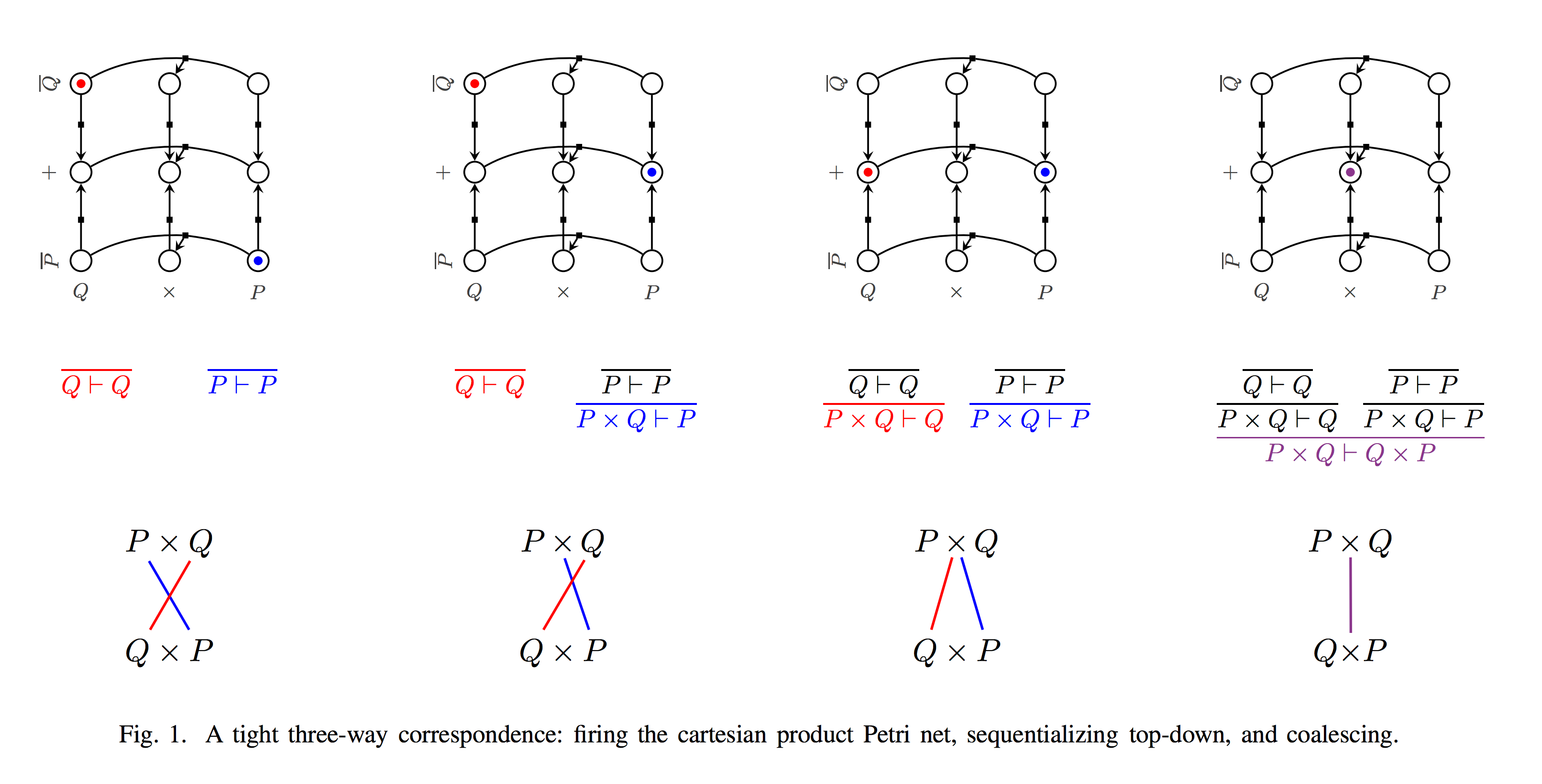### Dominic Hughes - Selected Papers

Complexity Bounds for Sum-Product Logic via Proof Nets and Petri Nets [pdf ps.gz bibtex]
With Willem Heijltjes
Logic in Computer Science, 2015.

We investigate efficient algorithms for the additive fragment of linear logic. This logic is an internal language for categories with finite sums and products, and describes concurrent two-player games of finite choice. In the context of session types, typing disciplines for communication along channels, the logic describes the communication of finite choice along a single channel.

We give a simple linear time correctness criterion for unit-free propositional additive proof nets via a natural construction on Petri nets. This is an essential ingredient to linear time complexity of the second author's combinatorial proofs for classical logic.

For full propositional additive linear logic, including the units, we give a proof search algorithm that is linear-time in the product of the source and target formula, and an algorithm for proof net correctness that is of the same time complexity. We prove that proof search in first-order additive linear logic is NP-complete.

Keywords: linear logic; proof complexity; Petri nets; sum-product categories; additive linear logic